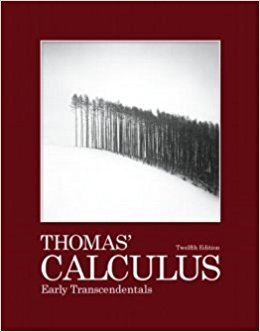×
×

# Solutions for Chapter 5: Integration## Full solutions for Thomas' Calculus Early Transcendentals | 12th Edition

ISBN: 9780321588760Solutions for Chapter 5: Integration

Solutions for Chapter 5
4 5 0 258 Reviews
24
5
##### ISBN: 9780321588760

This textbook survival guide was created for the textbook: Thomas' Calculus Early Transcendentals, edition: 12. Since 134 problems in chapter 5: Integration have been answered, more than 7997 students have viewed full step-by-step solutions from this chapter. Thomas' Calculus Early Transcendentals was written by and is associated to the ISBN: 9780321588760. This expansive textbook survival guide covers the following chapters and their solutions. Chapter 5: Integration includes 134 full step-by-step solutions.

Key Calculus Terms and definitions covered in this textbook
• Absolute value of a vector

See Magnitude of a vector.

• Acceleration due to gravity

g ? 32 ft/sec2 ? 9.8 m/sec

• Arccotangent function

See Inverse cotangent function.

• Bar chart

A rectangular graphical display of categorical data.

• Binomial probability

In an experiment with two possible outcomes, the probability of one outcome occurring k times in n independent trials is P1E2 = n!k!1n - k2!pk11 - p) n-k where p is the probability of the outcome occurring once

• Inverse cosecant function

The function y = csc-1 x

• Mathematical model

A mathematical structure that approximates phenomena for the purpose of studying or predicting their behavior

• Measure of center

A measure of the typical, middle, or average value for a data set

• Multiplicity

The multiplicity of a zero c of a polynomial ƒ(x) of degree n > 0 is the number of times the factor (x - c) (x - z 2) Á (x - z n)

• Negative numbers

Real numbers shown to the left of the origin on a number line.

• Outcomes

The various possible results of an experiment.

• Positive linear correlation

See Linear correlation.

• Range of a function

The set of all output values corresponding to elements in the domain.

• Rational zeros theorem

A procedure for finding the possible rational zeros of a polynomial.

• Rigid transformation

A transformation that leaves the basic shape of a graph unchanged.

• Transverse axis

The line segment whose endpoints are the vertices of a hyperbola.

• Union of two sets A and B

The set of all elements that belong to A or B or both.

• Vertical translation

A shift of a graph up or down.

• Weights

See Weighted mean.

• Zoom out

A procedure of a graphing utility used to view more of the coordinate plane (used, for example, to find theend behavior of a function).

×